# A Step-by-Step Guide to Python Float()

Updated:

Published:

The Python programming language uses various data types to store values, and float is one of the most critical data types every Python programmer should understand.The data type of a value will determine how Python can manipulate it. For example, it’s impossible to run a string function on an integer or a mathematical function on a string. As a programmer, you need to know the difference between the different data types and how to convert between data types when necessary.

This article will discuss the basics of float and how to use the float() method. We’ll also show you examples that use the float() method to convert different data types to floating-point numbers.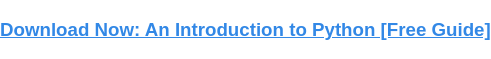Float values in Python are represented as 64-bit double-precision values. 1.8 x 10308 is the maximum value for any floating-point number. If the value inputted exceeds this max value, the Python program will return an error.

## Basic Data Types in Python

There are three main data types in Python: integers, strings, and floats.

### Integers

Any numerical value entered into a Python compiler will automatically be seen as a number, so there’s no need to declare that the value is a number. When numerical values are entered without decimals, Python sees them as integers.

Integers are whole numbers, positive or negative, without decimals and are of unlimited length. For example, 123 is an integer. So the program print(3) gives an output of 3. In Python, int is the underlying type of a Python integer.

Also, there’s no limit to how long an integer value can be in Python. The only constraint is your computer’s memory — beyond that, an integer can be as long as you need.

### Strings

A string is a collection of characters, which can include letters, numerals, and/or other symbols. In other words, a string is a sequence of character data. The string type is called str.

In Python, strings are enclosed in quotation marks, so 123 is considered an integer, and “123” is considered a string. Note that a string literal may also be delimited using single quotes. So to Python, “123” and 123 are both strings.

The code snippet below shows what happens when you enclose characters in either double or single quotation marks.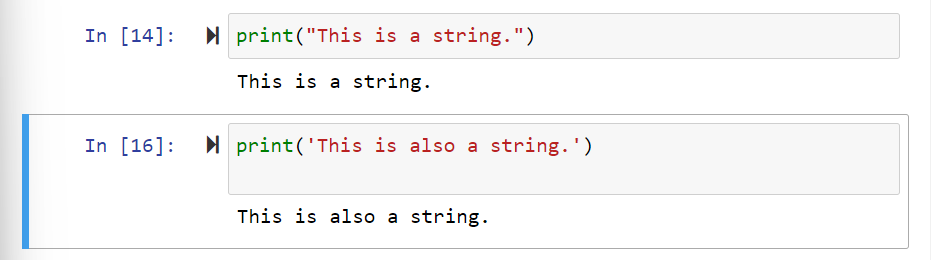A string in Python can contain as many characters as you need. Like with integers, the only limit is your computer’s memory capacity. A string can also be empty, (i.e., “”).

### Floats

Floats are values with decimals. For example, 1.23 is a float. If no value or a blank value is passed, the program will return 0.0 as the floating-point output.

You can also use the e or E characters followed by a positive or negative integer to specify scientific notation.

Both integers and floats are numerical data, which means we can carry out mathematical functions on them. We can add, subtract, multiply, or divide two floats together using Python.

For example, the program:

will give the output 6.0.

For a video explanation of floats and integers, check out this tutorial:

With this knowledge, let’s now learn how to use the Python float() function.

## How to Use the Python Float Function

To use the float() function, you first need to know its syntax and parameters.

### Float Syntax

In computer programming, syntax refers to rules that control the structure of symbols used in a programming language. If you don’t follow the rules of Python’s syntax, your code won’t be understood by the compiler.

As with every other function in python, float() has its syntax. The syntax for the float() function is:

float(value)

Here, value is an input parameter passed to the function when it’s called in the program or the element that should be converted. This parameter is optional and has a default value of 0.0.

### Float Parameters

A major advantage of Python over many other programming languages is that it has rich predefined libraries with different functions defined in them. Thus, using them is just a matter of calling the function.

However, when calling a function, it's important that the appropriate parameters are passed to the function. Parameters refer to the input arguments given to the function.

For float(), the parameter or value can be a regular integer or a string with decimal points. The value can also be empty.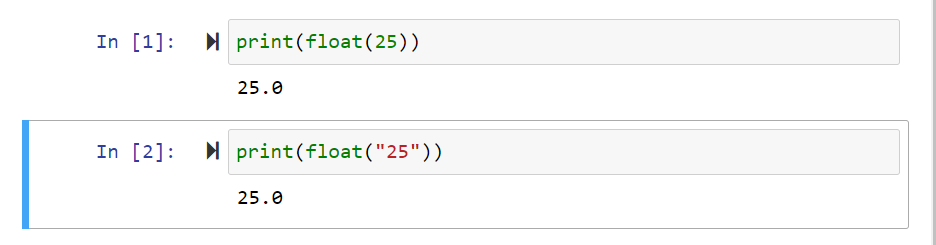The values that the float() method can return are:

• An equivalent floating-point number is returned for an argument that's passed.
• If no argument is passed or no value is provided, float() returns 0.0.
• If a string that’s not a floating-point number is passed, or the argument doesn’t match any of the cases mentioned in the two preceding points, float() returns an error.
• If the number exceeds the range of Python’s float, float() returns an OverflowError.

## Float Python Examples

We said the float() function returns different outputs depending on the value provided as parameters. Let’s now see some examples of the function in action.

### Example 1: Integers

Here’s what the float() function returns for an integer 123: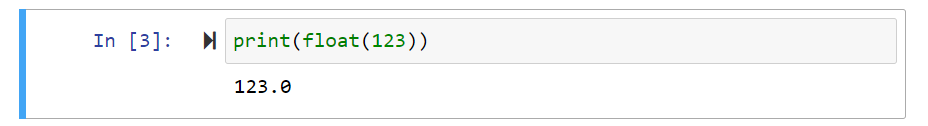Using the float() function, we’ve successfully converted the integer 123 to the floating-point number 123.0.

### Example 2: Decimals

Here’s what float() returns for the decimal value 1.23: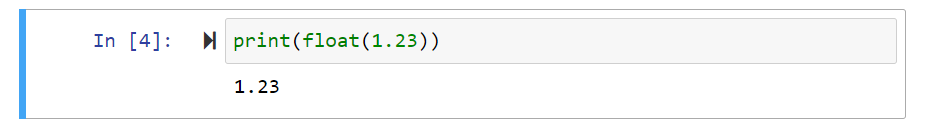### Example 3: String

This is what you get when the parameter is a string value, like “123”: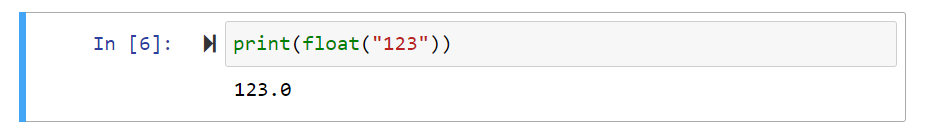In this example, the value “123” was stored as a string in the program. The float() function converted this value into a float. You can do the same thing with negative string values, too.

Keep in mind that the string must contain only numbers. If you try a string with characters other than numbers, the program will return an error.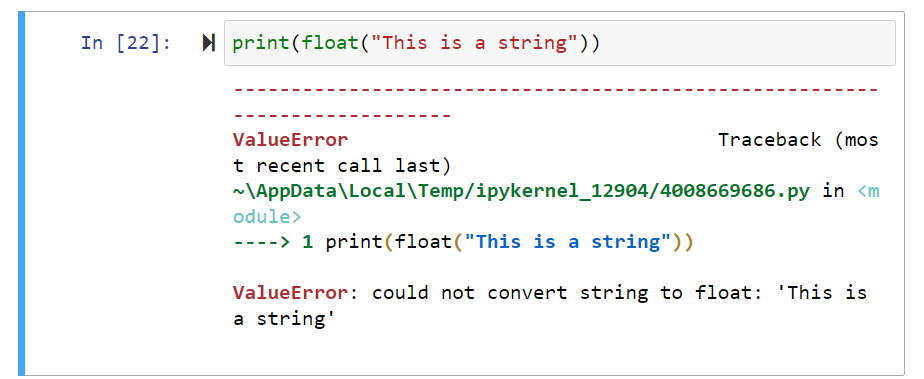### Example 4: String with White Spaces

The float() function returns a floating-point value for numerical strings with white spaces. For example, the string “      1.2” returns 1.2 as seen in the snippet below.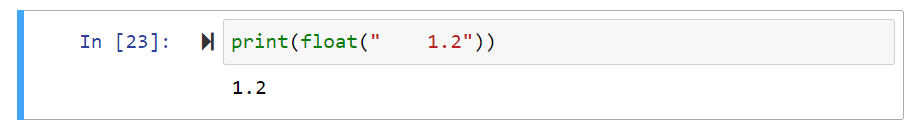### Example 5: Negative Integer

This is what you get when the parameter is a negative integer value like -123: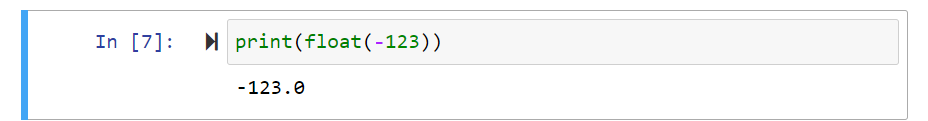Our code returns -123.0. Notice that the decimal point indicates that the value is now stored as a float instead of an integer.

### Example 6: Negative Decimals

If your argument is a negative decimal, the program will return the negative equivalent negative floating-point number.

For example, the input below gives an output of -1.2.### Example 7: Infinity

Infinity is denoted by inf in Python. It can either be negative infinity (-inf) or positive infinity (inf).

If the parameter is an integer that exceeds the maximum value of the Python floating-point number, then inf is returned: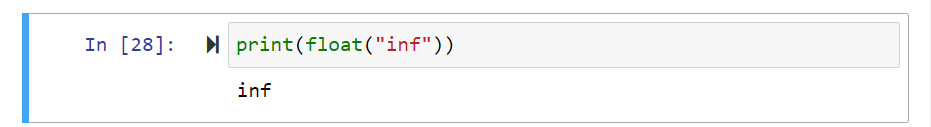The same output is returned if the parameter is “inf”, “Infinity”, “iNfiNity”, or any other variation since the parameter isn’t case-sensitive.

### Example 8: NaN

NaN stands for “Not a Number.” NaN represents the missing values in a dataset, and data scientists use it as a placeholder to represent undefined or missing values.

When passed the string “NaN” as a parameter, the float() function returns nan.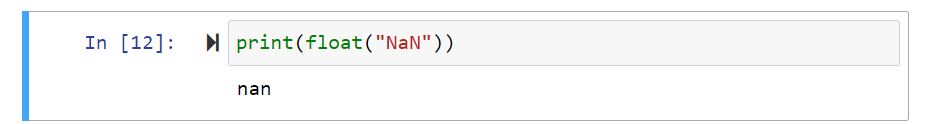Note that NaN is also not case-sensitive. Thus, passing Nan, NAN, nan, or any other variation will give you the same thing.

In addition, keep in mind that inf and NaN can only be defined by the float type. They cannot be created with the float() function.

## Using the Float Function in Python

As we’ve shown, float() is a key method that is handy for representing floating-point numbers. They allow programmers to work with decimal values, thus aiding specificity.

This article discussed the basics of Python’s float function and showed some examples of how to use this method to convert integers and strings to floats. At this point, it should be pretty straightforward to start working with floats!Topics: What Is Python?

## Related Articles

We're committed to your privacy. HubSpot uses the information you provide to us to contact you about our relevant content, products, and services. You may unsubscribe from these communications at any time. For more information, check out our Privacy Policy.

A guide for marketers, developers, and data analysts.